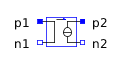CAD Geometry - MapleSim Help

Display geometry imported from an .stl file in your 3-D modelDescription To display CAD geometry, you add a copy of this component in the model workspace, attach an .stl file to your model, and then associate the CAD Geometry component with the .stl file by setting the Image Attachment parameter. The following image shows a model depicting a catapult with .stl geometry.For more information, see Adding Attached Shapes From an External File.Connections

 Name Description Modelica ID frame_a Connect this frame to the component or connection line for which you want to display the .stl geometry. frame_a frame_CoM Connect to this frame to gain access to the location of the center of mass. frame_CoMParameters

Name

Default

Units

Description

Modelica ID

Image Attachment

The .stl file that you want to display in your 3-D model. This drop-down list contains the names of the .stl files attached to the current model.

Image

Translation Offset

[0,0,0]

m

Offset between the origin of the .stl file and the frame to which it is attached. You can specify a value for this parameter to position the .stl geometry.

Note: The value of the translation offset will not affect the value of the inertia at the center of mass.

InitPos

${\mathrm{Type}}_{\mathrm{θ}}$

Sequence of body-fixed rotations used to describe the offset between the origin of the .stl file and the frame to which it is attached. You can specify a value for this parameter to position the .stl geometry.

RotType

${\stackrel{&conjugate0;}{\theta }}_{0}$

Rotational offset between the origin of the .stl file and the frame to which it is attached. You can specify a value for this parameter to position the .stl geometry.

InitAng

Scale Factor

1

m

The scale of the imported geometry.

Note: You must specify a numeric value. Custom values defined in parameter blocks, global parameters, or subsystem parameters cannot be used.

scale

color

red

Color of the geometry. For information on using the color selection tools, see Selecting Colors.

color

Dynamic ColorSelect this option to enable the Dynamic Color port. With the Dynamic Color port enabled, you can use the Color Blend component to change color throughout the simulation.

isInputColor

TransparentSelect this option to make the geometry component transparent.

transparent

SmoothWhen true, adjacent face geometry will appear smooth if their normals are less than or equal to the smooth factor angle.

isSmoothed

Smoothing Angle

15

deg

Angle in degrees when the smoothing algorithm should be applied to adjoining faces.

smoothAngle

Enable InertiaWhen checked, the calculated inertial properties are added to the geometry and the port, frame_CoM becomes enabled on the CAD component icon.

useInertia

ρ

1

$\frac{\mathrm{kg}}{{m}^{3}}$

Mass density.

rho

Mass

0

kg

The mass of the object. This value is calculated from the value provided in the mass density field, along with the volume specified in the .stl file.

Notes:

 • The accuracy of the calculated mass is dependent on tessellation of .stl export.
 • The mass density used in the calculation of the mass is assumed to be constant.

Mass

Inertia





$\left[\begin{array}{rrr}0& 0& 0\\ 0& 0& 0\\ 0& 0& 0\end{array}\right]$

$\mathrm{kg}\cdot {m}^{2}$

The inertia of the object at the center of mass. Similar to Mass, the inertia is calculated from the value provided in the mass density field.

Notes:

 • The accuracy of the calculated inertia value is dependent on tessellation of .stl export.
 • The mass density used in the calculation of the inertia at the center of mass is assumed to be constant.

Inertia

Center of Mass

$\left[\begin{array}{ccc}0& 0& 0\end{array}\right]$

m

Center of mass value provided in attached .stl file.

CoMPosUsing the CAD Geometry component with Inertial Properties When you check Enable Inertia, the values for mass density, mass, inertia, center of mass, and volume associated with the CAD Geometry component are used in the simulation. As an example, consider a model of an object with specified mass and inertia, rotating about the z-axis, constructed with  Fixed Frame, Revolute, and CAD Geometry components.With Enable Inertia selected this becomes analogous to a similar model built with Fixed Frame, Revolute, Rigid Body, and Rigid Body Frame components.This is important to note, because if your model already contains inertia properties through the inclusion of a Rigid Body/Rigid Body Frame assembly,selecting Enable Inertia will result in these inertial properties being added again to your model (essentially from a modeling standpoint you are adding a duplicate Rigid Body/Rigid Body Frame assembly).See Also